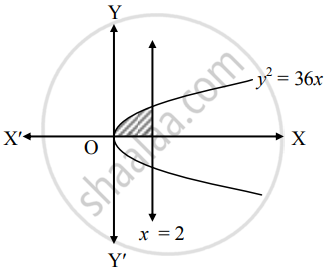# Find the area bounded by the curve y2 = 36x, the line x = 2 in first quadrant - Mathematics and Statistics

Sum

Find the area bounded by the curve y2 = 36x, the line x = 2 in first quadrant

#### Solution

Given equation of the curve is y2 = 36x

∴ y = +-  sqrt(36x)

∴ y = 6sqrt(x)     .....[∵ In first quadrant, y > 0]Required area = int_0^2 y  "d"x

= int_0^2 6sqrt(x)  "d"x

= 6[(x^(3/2))/(3/2)]_0^2

= 4[(2)^(3/2) - 0]

= 4(2sqrt(2))

= 8sqrt(2) sq.units

Concept: Area Bounded by the Curve, Axis and Line
Is there an error in this question or solution?